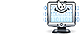Unit Conversion Software Web Widgets Loan Calculator Currency Rates Country Flags Unit Converter
 Faq HelpHelpContact
How to use unit converters

There are two types of unit converters: compact style and expanded style. The compact-style converter converts one unit of measurement into another unit of measurement while the expanded-style converter converts one unit of measurement into several different units of measurement.

How to use the compact style converter

Select the unit to convert from in the input units list, input its value in the corresponding input box and select the unit to Converter to in the output units list. The conversion result will immediately appear in the output box and in the results box.

How to use the expanded style converter

Choose the unit to convert from and type the value (for example, "1"). Our conversion engine will return a list of other units with the appropriate converted values.

You can sort the units alphabetically or by popularity, switch between compact and expanded view using the converter control panel which is at the top of each converter specific page, just below the site navigation panel.

Operations

Our converters support the use of mathematical expressions as well as simple values as input data for conversion. The following is a lists of supported basic operators, advanced operators, and constants.

The following table lists basic functions built into the converters:

 Operator Meaning Example + addition 12+34 - subtraction 5.6-7.8 * multiplication 5.5*110 / division 128/5 % modulo (finds the remainder after division) 14%2 () Forces the expression within the parentheses to be calculated first, overriding the normal order of operations 2*(3+4)

The following table lists advanced functions and constants built into the converters:

 Operator Meaning Example pow exponentiation (raise to a power of) pow(2,8) sqrt square root sqrt(16) sin, cos, tan trigonometric functions (numbers are assumed to be radians) cos(pi/3) asin, acos, atan, atan2 inverse trigonometric functions acos(.5) log logarithm base e log(16) exp e raised to a power exp(4.1) abs the absolute value abs(-5) round round to the nearest integer round(1.45) ceil the smallest integer greater than or equal to ceil(4.95) floor the greatest integer less than or equal to floor(4.05) max the greater number max(0,1,2,3,4) min the lesser number min(5,6,7,8,9) Constant Meaning Example e mathematical constant e, the base of natural logarithms, approximately 2.718 e pi the ratio of the circumference of a circle to its diameter, approximately 3.141592653589793 pi ln2 the natural logarithm of 2, approximately 0.693 ln2 ln10 the natural logarithm of 10, approximately 2.302 ln10 log2e the base-2 logarithm of e, approximately 1.442 log2e log10e the base-10 logarithm of e, approximately 0.434 log10e sqrt1_2 the square root of 0.5, or one divided by the square root of 2, approximately 0.707 sqrt1_2 sqrt2 the square root of 2 sqrt2Copyright © 1998-2009 UnitConversion.org Privacy & Terms | About | Faq | Help | Contact | Link to Us